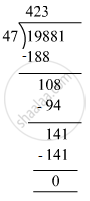# Divide and check your answer by the corresponding multiplication in the following: 19881 ​÷ 47 - Mathematics

Fill in the Blanks

Divide and check your answer by the corresponding multiplication in the following:

19881 ​÷ 47

#### SolutionDividend = 19881, Divisor = 47 , Quotient = 423, Remainder = 0
Check: Divisor ×Quotient + Remainder= 47 × 423 + 0
= 19881
=Dividend
Hence, Dividend = Divisor × Quotient + Remainder

Concept: Concept for Whole Numbers
Is there an error in this question or solution?

#### APPEARS IN

RS Aggarwal Class 6 Mathematics
Chapter 3 Whole Numbers
Exercise 3E | Q 1.2 | Page 56